Hostname: page-component-546b4f848f-bvkm5 Total loading time: 0 Render date: 2023-06-04T16:33:57.540Z Has data issue: false Feature Flags: { "useRatesEcommerce": true } hasContentIssue false

# McKay matrices for finite-dimensional Hopf algebras

Published online by Cambridge University Press:  08 February 2021

## Abstract

For a finite-dimensional Hopf algebra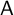$\mathsf {A}$ , the McKay matrix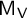$\mathsf {M}_{\mathsf {V}}$ of an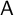$\mathsf {A}$ -module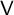$\mathsf {V}$ encodes the relations for tensoring the simple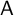$\mathsf {A}$ -modules with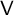$\mathsf {V}$ . We prove results about the eigenvalues and the right and left (generalized) eigenvectors of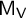$\mathsf {M}_{\mathsf {V}}$ by relating them to characters. We show how the projective McKay matrix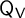$\mathsf {Q}_{\mathsf {V}}$ obtained by tensoring the projective indecomposable modules of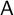$\mathsf {A}$ with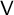$\mathsf {V}$ is related to the McKay matrix of the dual module of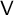$\mathsf {V}$ . We illustrate these results for the Drinfeld double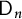$\mathsf {D}_n$ of the Taft algebra by deriving expressions for the eigenvalues and eigenvectors of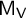$\mathsf {M}_{\mathsf {V}}$ and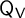$\mathsf {Q}_{\mathsf {V}}$ in terms of several kinds of Chebyshev polynomials. For the matrix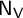$\mathsf {N}_{\mathsf {V}}$ that encodes the fusion rules for tensoring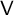$\mathsf {V}$ with a basis of projective indecomposable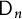$\mathsf {D}_n$ -modules for the image of the Cartan map, we show that the eigenvalues and eigenvectors also have such Chebyshev expressions.

## MSC classification

Type
Article
Information
Canadian Journal of Mathematics , June 2022 , pp. 686 - 731

## Access options

Get access to the full version of this content by using one of the access options below. (Log in options will check for institutional or personal access. Content may require purchase if you do not have access.)

## References

Agerholm, T. and Mazorchuk, V., On selfadjoint functors satisfying polynomial relations . J. Algebra 330(2011), 448467.CrossRefGoogle Scholar
Benkart, G., Biswal, R., Kirkman, E., Nguyen, V. C., and Zhu, J., Tensor representations for the Drinfeld double of the Taft algebra. Preprint, 2020. arXiv#2012.1527 Google Scholar
Benkart, G., Diaconis, P., Liebeck, M., and Tiep, P., Tensor product Markov chains . J. Algebra 561(2020), 1783.CrossRefGoogle Scholar
Chen, H. X., A class of noncommutative and noncocommutative Hopf algebras: the quantum version . Comm. Algebra 27(1999), no. 10, 50115032.CrossRefGoogle Scholar
Chen, H. X., Irreducible representations of a class of quantum doubles . J. Algebra 225(2000), 391409.CrossRefGoogle Scholar
Chen, H. X., Finite-dimensional representations of a quantum double . J. Algebra 251(2002), 751789.CrossRefGoogle Scholar
Chen, H. X., Representations of a class of Drinfeld doubles . Comm. Algebra 33(2005), 28092825.CrossRefGoogle Scholar
Chen, H. X., Mohammed, H. S. E., and Sun, H., Indecomposable decomposition of tensor products of modules over Drinfeld doubles of Taft algebras . J. Pure Appl. Algebra 221(2017), no. 11, 27522790.CrossRefGoogle Scholar
Cohen, M. and Westreich, S., Characters and a Verlinde-type formula for symmetric Hopf algebras . J. Algebra 320(2008), no. 12, 43004316.CrossRefGoogle Scholar
Cohen, M. and Westreich, S., Higman ideals and Verlinde-type formulas for Hopf algebras . In: Ring and module theory, Trends Math., Birkhäuser/Springer Basel AG, Basel, 2010, pp. 91114.CrossRefGoogle Scholar
Cohen, M. and Westreich, S., Structure constants related to symmetric Hopf algebras . J. Algebra 324(2010), no. 11, 32193240.CrossRefGoogle Scholar
Curtis, C. W. and Reiner, I., Methods of representation theory. Vol. I. With applications to finite groups and orders. Reprint of the 1981 original. Wiley Classics Library. A Wiley-Interscience Publication, John Wiley & Sons, Inc., New York, 1990.Google Scholar
Erdélyi, A., Magnus, W., Oberhettinger, F., and Tricomi, F.G., Higher transcendental functions. Vol. II. Based on notes left by Harry Bateman. Reprint of the 1953 original, Robert E. Krieger Publishing Co., Inc., 1981.Google Scholar
Etingof, P., Gelaki, S., Nikshych, D., and Ostrik, V., Tensor categories . Mathematical Surveys and Monographs, 205, American Mathematical Society, Providence, RI, 2015.CrossRefGoogle Scholar
Etingof, P., Golberg, O., Hensel, S., Liu, T., Schwendner, A., Vaintrob, D., and Yudovina, E., Introduction to representation theory . Student Mathematical Library, 59, American Mathematical Society, Providence, RI, 2011.CrossRefGoogle Scholar
Etingof, P., Nikshych, D., and Ostrik, V., On fusion categories . Ann. Math. 162(2005), no. 2, 581642.CrossRefGoogle Scholar
Fulton, W. and Harris, J., Representation theory. A first course . Graduate Texts in Mathematics, 129, Readings in Mathematics, Springer-Verlag, New York, 1991.Google Scholar
Geer, N., Kujawa, J., and Patureau-Mirand, B., Ambidextrous objects and trace functions for nonsemisimple categories . Proc. Amer. Math. Soc. 141(2013), no. 9, 29632978.CrossRefGoogle Scholar
Grinberg, D., Huang, J., and Reiner, V., Critical groups for Hopf algebra modules . Math. Proc. Cambridge Philos. Soc. 168(2020), no. 3, 473503.CrossRefGoogle Scholar
Hennings, M. A., Invariants of links and 3-manifolds obtained from Hopf algebras . J. Lond. Math. Soc. 54(1996), 594624.CrossRefGoogle Scholar
Jedwab, A., A trace-like invariant for representations of Hopf algebras . Comm. Algebra 38(2010), no. 9, 34563468.CrossRefGoogle Scholar
Kac, G. I. and Palyutkin, V. G., Finite ring groups . Trudy Moskov. Mat. Obsc. 15(1966), 224261.Google Scholar
Kashina, Y., Semisimple Hopf algebras of dimension 16 . J. Algebra 232(2000), 617663.CrossRefGoogle Scholar
Kauffman, L. and Radford, D., A necessary and sufficient condition for a finite-dimensional Drinfeld double to be a ribbon Hopf algebra . J. Algebra 159(1993), no. 1, 98114.CrossRefGoogle Scholar
Lorenz, M., Representations of finite-dimensional Hopf algebras . J. Algebra 188(1997), 476505.CrossRefGoogle Scholar
Lorenz, M., A tour of representation theory . Graduate Studies in Mathematics, 193, American Math. Society, Providence, RI, 2018.CrossRefGoogle Scholar
Mason, J. C. and Handscomb, D. C., Chebyshev polynomials . Chapman & Hall/CRC, Boca Raton, FL, 2003.Google Scholar
Mazorchuk, V. and Miemietz, V., Transitive 2-representations of finitary 2-categories . Trans. Amer. Math. Soc. 368(2016), no. 11, 76237644.CrossRefGoogle Scholar
McKay, J., Graphs, singularities, and finite groups . In: The Santa Cruz Conference on Finite Groups (Univ. California, Santa Cruz, Calif., 1979), Proc. Sympos. Pure Math., 37, Amer. Math. Soc., Providence, RI, 1980, pp. 183186.Google Scholar
NIST Digital Library of Mathematical Functions. https://dlmf.nist.gov/ Google Scholar
Oberst, U. and Schneider, H. J., Über Untergruppen endlicher algebraischer Gruppen . Manuscripta Math. 8(1973), 217241.CrossRefGoogle Scholar
Radford, D., Hopf algebras, Series on Knots and Everything , 49, World Scientific Publishing Co., Hackensack, NJ, 2012.Google Scholar
Shimizu, K., The monoidal center and the character algebra . J. Pure Appl. Algebra 221(2017), no. 9, 23382371.CrossRefGoogle Scholar
Steinberg, R., Finite subgroups of SU2, Dynkin diagrams and affine Coxeter elements . Pac. J. Math. 118(1985), no. 2, 587598.CrossRefGoogle Scholar
Sun, H., Mohammed, H. S. E., Lin, W., and Chen, H. X., Green rings of Drinfeld doubles of Taft algebras . Comm. Algebra 48(2020), no. 9, 39333947.CrossRefGoogle Scholar
Verlinde, E., Fusion rules and modular transformations in 2D conformal field theory . Nucl. Phys. B 300(1988), no. 3, 360376.CrossRefGoogle Scholar
Witherspoon, S. J., The representation ring and the centre of a Hopf algebra . Canad. J. Math. 51(1999), no. 4, 881896.CrossRefGoogle Scholar
Zhang, Y., Wu, F., Liu, L., and Chen, H. X., Grothendieck groups of a class of Drinfeld doubles . Alg. Colloq. 15(2008), no. 3, 431448.CrossRefGoogle Scholar
Zhu, Y., Hopf algebras of prime dimension . Int. Math. Res. Not. 1(1994), 5359.CrossRefGoogle Scholar